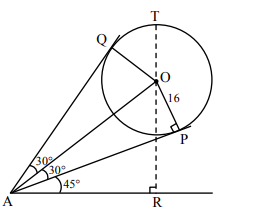# A spherical gas balloon of radius 16 meter subtends`
Question:

A spherical gas balloon of radius 16 meter subtends an angle $60^{\circ}$ at the eye of the observer $\mathrm{A}$ while the angle of elevation of its center from the eye of $\mathrm{A}$ is $75^{\circ}$. Then the height (in meter) of the top most point of the balloon from the level of the

1. $8(2+2 \sqrt{3}+\sqrt{2})$

2. $8(\sqrt{6}+\sqrt{2}+2)$

3. $8(\sqrt{2}+2+\sqrt{3})$

4. $8(\sqrt{6}-\sqrt{2}+2)$

Correct Option: , 2

Solution:$\mathrm{O} \rightarrow$ centre of sphere

$\mathrm{P}, \mathrm{Q} \rightarrow$ point of contact of tangents from $\mathrm{A}$

Let $T$ be top most point of balloon \& $R$ be foot of perpendicular from $\mathrm{O}$ to ground.

From triangle $\mathrm{OAP}, \mathrm{OA}=16 \operatorname{cosec} 30^{\circ}=32$

From triangle $\mathrm{ABO}, \mathrm{OR}=\mathrm{OA} \sin 75^{\circ}=32 \frac{(\sqrt{3}+1)}{2 \sqrt{2}}$

So level of top most point $=$ OR $+$ OT

$=8(\sqrt{6}+\sqrt{2}+2)$